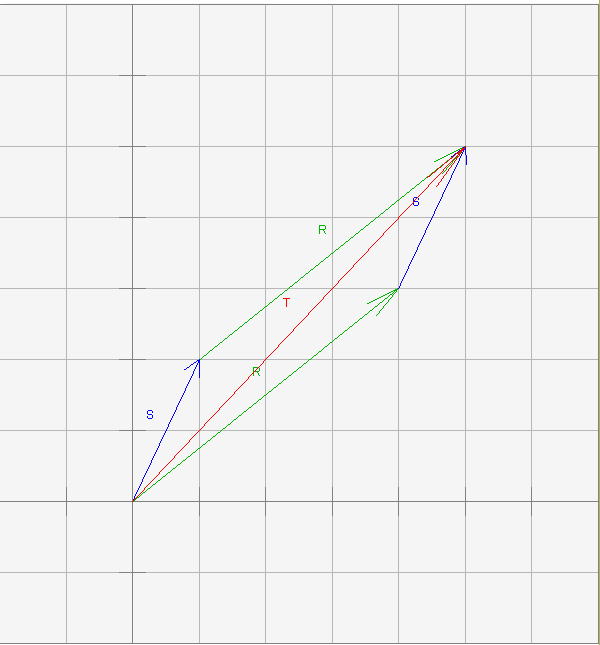As before,

```T = (5, 5)T
```

# Parallelogram for Vector AdditionThere are two ways to form the sum:

```T = S + R
T = R + S
```

where

```R = ( 4, 3 )T
S = ( 1, 2 )T
```

There are two ways to draw the diagram, depending on which arrow's tail you put at the origin. If you draw both versions, then you get a parallelogram with the sum of the vectors as the diagonal arrow whose tail starts at the origin.

What is a parallelogram? you might ask, if your high school geometry is a bit murky. A parallelogram is a four sided figure with opposite sides parallel and equal in length. So, for example, the blue arrows representing the vector s are the same length and same direction. The green arrows representing the vector r have their same length and same direction.

### QUESTION 15:

u = ( -3, 2 )T,     v = ( 1, -5 )T     form the sum:     w = u + v